﻿ Prediction of Water Turbidity using Artificial Neural Networks: A Case Study of Kiriku-Kiende Settling Basin in Embu County, KenyaPublications are Open
Access in this journal
Article Versions
Export Article
• Normal Style
• MLA Style
• APA Style
• Chicago Style
Research Article
Open Access Peer-reviewed

### Prediction of Water Turbidity using Artificial Neural Networks: A Case Study of Kiriku-Kiende Settling Basin in Embu County, Kenya

Patrick N. Namu , James M. Raude, Benedict M. Mutua, Raphael M. Wambua
American Journal of Water Resources. 2017, 5(3), 54-62. DOI: 10.12691/ajwr-5-3-1
Published online: July 13, 2017

### Abstract

This paper presents the use of Artificial neural networks (ANN) as a viable means of predicting water turbidity. This was based on the experimental data collected from a physical settling basin model, which was set up at the Civil Engineering Department in Jomo Kenyatta University of Agriculture and Technology. High turbid water has contributed to low water application efficiencies in Kiriku-Kiende project. Lack of adequate detention time for sediment settlement in the basin has resulted to sediment being released in to irrigation network. To have an optimal settling basin design, then prediction of hourly water turbidity is paramount. The turbidity of the water at the inlet and outlet of the settling basin in Kiriku-Kiende irrigation project in Embu Sub-County was determined. Samples collected were analyzed and more data collected from the physical model. Two variables (flow rate and settling Time) were used in this analysis as input variables and water turbidity as output variable. Four hundred ANN models were developed out of which four best models were used to identify the most effective model. Sensitivity analysis (SA) was carried out by using leave one out approach to assess the effect of the parameters of ANN on the prediction of turbidity of raw water in a water-settling basin. The ANNs developed were successfully trained and tested using the experimental data sets and the performance of ANNs models were determined using various statistical measures. Results show that using the five statistical measures, the ANN-PN204 with a nomenclature of 1-9-1 gave the best prediction for water turbidity. This demonstrates that ANN is capable of modeling hourly turbidity levels with good accuracy when proper variables, their previous time step on sediment settling and their discharges are used as inputs of networks.

### 1. Introduction

Irrigation schemes are usually designed and implemented based on assumed efficiencies in water conveyance, water distribution and water applications. However, over time, physical changes in the schemes such as poor maintenance of structures, soil property changes and deterioration of equipment may alter these efficiencies 1.

Kiriku-Kiende irrigation project abstracts its water from Kirurumwe River. Additionally, water from River Kirurumwe is vital for water supply purposes to the surrounding towns. Kirurumwe River basin is characterized by loose red soil and a steep slope. Therefore, during rainy season there is a lot of soil erosion. This soil is carried into Kirurumwe River which makes the river highly turbid with turbidity of 464 Nephelometric turbidity units (NTU) being recorded. Sediment yield and turbidity are serious problems being experienced by the project management in the area 2. Moreover, Irrigation induced soil erosion has been observed to pose challenges in other places in the world. In the North western United States, a number of studies measured annual sediment yields from furrow-irrigated fields and it was found that the amount of sediment per year was 20 t/ha with some fields exceeding 100 t/ha per year 3. For example, in one study of 42 reservoirs in Iowa, Nebraska, and Missouri, 18 reservoirs lost 25 % of their storage capacity in 11 years 4, 5. Further, the Yellow River that is the second largest River in China is known for its high sediment discharge with an average value of 1.6 x 109 tons/year 6. 7 approximated that 1% of the world’s water storage capacity in reservoirs is lost every year through sediment deposition.

Locally, a study conducted in Murang’a county in Kenya by 1 on smallholder irrigation projects, sprinkler clogging caused by sediments resulted to low coefficients of uniformity in water application. Gem-Rae irrigation scheme in Kisumu-Kenya that was commissioned in 1985 had to be abandoned after 12 years of operation due to clogging of intake and main canals by sediment. In 1997 approximately 200m3 of sediment were removed weekly from the intake of this project 8. Clogging and blocking of irrigation structures due to sediment have contributed to low overall irrigation water delivery and high maintenance cost. At the same time, causing reduced capacity of water transport and annual irrigation acreage reduction

### 2. Artificial Neural Networks

Artificial neural networks (ANN) are a form of computing that simulate the operation of human brain and nervous system 9. Although the concept of artificial neurons was first introduced in 1943 10, research into applications of ANNs has blossomed since the introduction of the back-propagation training algorithm for feed-forward ANNs in 1986 11, 12. One of the advantages of the ANN modeling technique is that the definition of physical processes need not to be done 9, 13, this property makes it appropriate in processing large and complex data sets including that of turbidity prediction.

Currently, Artificial Neural Networks have been increasingly employed in modeling of Engineering processes especially in water resources management and irrigation systems 14. This is due to their ability to give input-output relationship without any understanding of the physical process 15, 16. ANN is capable to model any arbitrary complex non-linear process that relates sediment load to continuous water discharge 17. It is a very practical and promising modeling tool in the context of sediment load prediction and its output can potentially be used in solving environmental problem including water resources modeling and management problems 14.

In addition 18 applied ANN to model the estimation of sediment load in an alluvial river in India. While 17 presented an ANN model to estimate the natural sediment discharge in rivers, which reflected that neural network models can be successfully applied in modeling sediment transport. River flow and sediment transport in a river system were modeled using ANN by 19 and it was concluded that ANN was a reliable model for describing flow and sediment transport processes in a river system. 20 used ANN to simulate the suspended sediment load in a river. 21 developed a feed forward back propagation ANN for computation of event based temporal variation of sediment yield from the watersheds. ANN models were developed to predict both runoff and sediment yield on a daily and weekly basis, for Upper Siwane River watershed in India 22.

2.1. Concept of ANN Model

The ANN model processes information through an elaborate network of neurons that are linked together. It simulates outputs based on certain inputs by a working principle resembling that of human brain. The neuron has four main inputs; the cell body, synapse, dendrites and axon. A neuron receives an input signal transmits it to the cell body where it is processed and then an output is produced. In a biological neuron, both the inputs and outputs are electrical signals. The input signals are normally passed to the neuron through dendrites while the output signal is released through a single channel called axon. On every dendrite contact point there is a part called synapse which works like a valve to open or close for signal flow. This synapse allows input signals to pass through or stops some signals based on the conditions of operation of the system 23.

The ANN model is similar to a biological neuron in that it has multiple input channels, data processing units and output channels called dendrites, cell body and axon respectively as represented in Figure 1. The input signals (X1, X2,…....Xp) are passed to the neuron through the dendrites that represent different input channels. Each channel has its own weight referred to as connection weight denoted as W1, W2, ……Wp. The weights are very critical since they allow for collection and processing of signals based on their magnitude and effects on input functions. If a weight function gives a non-zero value at the synapse, it is allowed to pass through the cell body. All the conveyed signals are normally integrated by summing up all the input 16, 24. The integration of signals is achieved by application of a mathematical model referred to as activation function, within the cell body to generate an output signal. According to 24, 25, the relationship between the input and output signal within an ANN model is the function given as;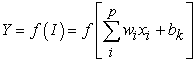(1)

Where Xi is the input signal I, Wi is weight attached to the input signal I, P is the number of input signals, bk is bias at the cell of the body. Y is the output, f is activation function. Numerous activation equations or functions can be used within the neurons. The most common functions used in the ANN models include; the step-function, non-linear sigmoid, hyperbolic tangent and linear activation functions 26, 27.

The main objective of the research presented in this paper was to use ANN in predicting turbidity at different lead times based on laboratory data collected from a physical settling basin model. This was achieved through data training whereby the ANN output of the network was compared with the measured turbidity values. ANN training was done through Levenbarg-Marquardt algorithm.

• Figure 1. Typical structure of Artificial Neural Network 
2.2. ANN Training

Training in ANNs is usually divided into supervised and unsupervised learning 29. In supervised learning, the network is presented with a historical set of model inputs and the corresponding (desired) outputs. The actual output of the network is compared with the desired output and an error is calculated. This error is used to adjust the connection weights between the model inputs and outputs to reduce the error between the historical outputs and those predicted by the ANN. The number of training samples presented between weight updates is called an epoch 30. In unsupervised learning, the network is only presented with the input stimuli and there are no desired outputs. The network itself adjusts the connection weights according to the input values 31.

The objective of the training process is to minimize the errors between the predicted and actual outputs 28, 31. This minimization process can be achieved by the error function with respect to all variables in the neural network (connection weights, network architecture, learning rateand threshold).

The choice of training rate is critical and the optimum training rate is usually determined by trial and error. If the learning rate is selected to be small, convergence will be achieved, however, it will be very slow. In addition, convergence will be subject to the local minimum in the error surface that is closest to the random starting position. On the other hand, if the learning rate is selected to be large, convergence will never occur 11, 32. Artificial Neural Networks (ANN) in MATLAB 2015a was used to build ANN models for predicting turbidity in a settling basin. This was eventually to optimize the hydraulic parameters resulting to ann optimal settling basin to settle discrete particles. Therefore, ANN was used to develop the relation between sediment settlement and different inflows by changing the different number of neurons in hidden layer. The best ANN is chosen as the predictive model on taking into account the different scenarios. ANN models are considered suitable for this application because longer-term predictions are required, non-linear relationships are suspected and it is difficult to prescribe the exact mathematical model.

### 3. Materials and Methods

3.1. The Study Area

The Kiruku-Kiende Irrigation project is located in Gaturi South in Nembure division of Embu West Sub-County in Kenya at coordinates 9949924N, 336052 E and with an average elevation of 1508 m above sea level. The scheme covers a gross area of 2,261.5 hectares with about 52 hectares being irrigable land. The water source for Kiruki Kiende Irrigation Project is Kirurumwe River in the Tana Sub-basin (See Figure 2). The River originates from the foot of Mt. Kenya near Manyatta with many tributaries along its reach. High flows occur in the months of April and low flows in the month of September. The project was chosen since its water originates from a highly turbid River in the county. Kirurumwe River basin is characterized by loose red soil and a steep slope and as such during rainy season there is a lot of soil erosion which is transported to this river.

• Figure 2. Map of the study area

Sediment yield and turbidity are serious problems being experienced by the project management in the area. Complains of sprinkler clogging have been reported at the County irrigation offices by scheme management.

The water samples from Kirurumwe River were used as the basis for preparing the constituent experimental samples. The turbidity of the water at the inlet and outlet of the settling basin in Kiriku-Kiende irrigation project in Embu Sub-County was determined. This was done so as to have water turbidity values for water before particles are settled and after settlement. This data was used to simulate the turbidity values for the physical model. Kiriku-Kiende settling basin has a length of 4.2 m, a breadth of 1.2 m and a height of 2.0 m. Samples collected were analyzed and more data collected from a physical model see Figure 3.

• Figure 3. The experimental set up
3.2. Data Collection

Using settling velocity for sand particle formula 33 and a tank sizing ratio of ratio 2:1 for linear measurements, the dimensions of physical model for settling basin were taken as Length of 2 m, breadth of 0.5 m and a height of 0.4 m. The inflow pipe used was of diameter 50 mm connected to a header tank at a height of 1.6 m with a measuring flow meter next to the control valve. This was used to run the various experiments (Figure 3). The study using the model was carried out in the Civil Engineering Laboratory at Jomo Kenyatta University of Agriculture and Technology (JKUAT).

Sand particles were prepared by sieving them through 0.20 mm diameter of sieving mesh. The sieved sand particles were then used to prepare turbid water in a 200 litre container. The turbidity for the water was measured, recorded and maintained between 390 to 470 Nephelometric turbidity units (NTU) to simulate turbidity in River Kirurumwe... The water flowed to the settling basin by gravity at an increasing flow velocity. The flow was regulated by a gate valve fixed next to a flow meter and was used in recording the flow rate. A time interval of 20 minutes was chosen, since this represented a time at which there was a notable change in turbidity. The water was drawn from the super surface of the settling basin and put in the measuring bottles. Water samples were taken at intervals of 20mins over a period of 300 minutes. After 300 minutes, the water in the settling tank was almost clear to a level acceptable for irrigation purposes. The collected samples from turbid water were then tested for level of turbidity using turbidity meter in the soil laboratory at the Soil, Water and Environmental Engineering Department (SWEED).

A total of six (6) different flow rates with turbidities ranging from 390 to 470 NTU (referred to as highly turbid water) were set, corresponding to 4.8 l/min, 5.7 l/min, 8.7 l/min, 9.9 l/min, 10.5 l/min and 11.1 l/min respectively. This process was repeated for lower turbidities ranging from 180 to 250 NTU for flow rates of 8.7 l/min, 9.9 l/min, 10.5 l/min and 11.1 l/min respectively also referred as low turbid water.

3.3. Predicting the Water Turbidity

The data collected was split into three where part one was used for training the model, part two for model validation and part three for analyzing and testing the model using the Artificial Neural Networks model. Measured turbidity values and settling time were set as input parameters whereas predicted turbidity was considered as system output. A data base consisting of 160 data sets obtained from the experimental set-up was used to develop the predictive model.

3.4. Performance Evaluation of the Models

In ANN the input and output variables used in the modelling were normalized by fitting their values between -1 and 1 to speed up the prediction process as stated by 34, 35. In the present study, the collected turbidity and time data values were scaled from minimum to maximum value by applying the following function: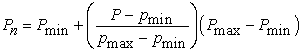(2)

Where;

Pn = the normalized parameter,

P = actual parameter,

Pmin = the selected minimum value for standardization,

Pmax = the selected maximum value for standardization

pmin = minimum of the actual parameters

pmax = maximum of the actual parameters.

About 70% equivalent to 112 out of 160 of the data sets were selected randomly by the model and set as training data. Then 15% (equivalent to12 data sets) for validation and another 12 data set for test purposes.

In this research, four statistical measures were used to determine the efficiency of the ANN models. These included the correlation coefficient (R), the Mean Square Error (MSE), Root Mean Square Error (RMSE) and the Mean Absolute Error (MAE)

The statistical relationship between the measured and the predicted data values for Kiriku-Kiende settling basin was done using the Correlation Coefficient (R). The fundamental function was customized to the respective data input and outputs of the following general form:(3)

Where;

R = correlation coefficient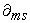= measured value of the turbidity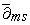= mean of the measured values of the turbidity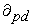= predicted value of the turbidity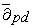= mean of the predicted values of the turbidity

n = number of data points considered

The R is a measure of the strength of the relation between measured and predicted turbidity values. It varies from 0 to 1. The values of 0 and 1 indicate a poor and perfect prediction capability of the model respectively.

The Mean Square Error (MSE) is a measure of the difference between the measured and predicted turbidity values from different flow rates. It measures the average of the squares of the errors between two values being compared. For the purpose of turbidity, the following function was adopted for calculating MSE associated with turbidity prediction: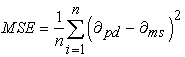(4)

Where;

MSE = mean square error= measured value of the turbidity= predicted value of the turbidity

n = number of data points.

The MSE ranges from 0 to 1. The smaller the MSE value the better the prediction capability of the model.

The mean absolute error (MAE) was determined from the relation: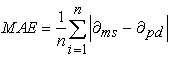(5)

Where;

MAE = the mean absolute error= measured value of the turbidity= predicted value of turbidity

n = number of data points

The MAE was used to measure the average magnitude of errors of the set of predicted values. The values of MAE increase from zero to large positive values. The higher the value of MAE, the higher the discrepancy between predicted and measured values 36, 37.

The Root Mean Square Error (RMSE) is a frequently used measure of the difference between values predicted by a model and the values actually observed from the environment that is being modelled 37, 38. The RMSE of the model prediction with respect to the predicted variable Xpd was defined as the square root of the mean squared error and was determined by function: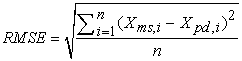(6)

Where;

Xms =is measured values and

Xpd =is predicted /modelled values at time i.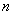=number of observations

The RMSE values were used to distinguish model performance in the calibration period with that of a validation period. The lower the RMSE value, the more accurate the prediction was 38.

3.4.1. Sensitivity Analysis

Prior to calibration of the ANN, a sensitivity analysis was performed to identify key calibration input variables. The parameter considered here are, training times, settling time and the number of neurons in the hidden layer. Each parameter was varied while the rest two were kept constant. This was repeated for each parameter while holding others constant. For every training the coefficient of determination for both training and validation parameters were recorded. Sensitivity index was then determined by function: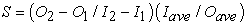(7)

Where S is the sensitivity index, I1 and I2 are the smallest and the largest input-values respectively; O1 and O2 are the model output values corresponding to I1 and I2 respectively. Iave and Oave are respectively the average I1 and I2 and the average of O1 and O2 9. The greater the value of S, the greater the effect an input parameter has on a particular output.

### 4. Results and Discussion

The results presented in Table 1 refer to the ANN that displayed the lowest error herein defined as the mean square error and the Nash–Sutcliffe Efficiency for every lead-time (20, 40, 60 and 80 minutes). In naming the models (e.g. PN 204), the first two letters (PN) refer to Predicted Network, then the first two numbers refer to lead-time that is 20, 40, 60 and 80 minutes lead time respectively. The last third number represented the flow rate where by 1, 2, 3, 4, 5,6 and 7 represents 4.8 l/min, 5.7 l/min, 8.7 l/min, 9 l/min, 9.9l/min, 10.5 and 11.1 l/min respectively. Table 1 show the four best ANN structures.

A bar graph of MSE, R, RMSE, and MAE versus their accuracy for the best test set of 112.was plotted (Figure 4) The values of MSE are increasing from 0.1246 to 0.9278. According to 37, 38, the lower the MSE the better is the long-term model prediction hence PN204 is the best model when MSE is considered. The bar graphs show a linear relationship where the RMSE is increases with increase in water flow rates. The minimum RMSE is often used as a criterion for selecting the better neural network. Based on this criterion, the network trained to 0.51 (PN-204) would be chosen as the better network.

• Figure 4. Comparison of quantitative measures of accuracy for the four ANN models

Figure 4 also shows results of MAE, where the error is increasing with increase in flow rate, which shows increase in discrepancy of the observed and predicted data. The best neural network chosen is the network with lower values of MAE, in this case training error of 0.13 corresponding to PN-204 model.

The four ANN models in Table 1 were used to develop the relation of sediment settlement and the flowrate for a discrete particle setting in a settling basin. The number of hidden neurons in each activation layer was selected by trial and error method. From the statistical analysis of each model above, the ANN model PN-204 is the best for predicting the water turbidity for irrigation purposes. This is because it has the least RMSE, MAE and MSE. On the correlation, PN-204 is almost the same with PN-402. However, PN-204 is a better choice since it has met most of the criteria. A strong correlation was also found between the observed and estimated sediment load values for the same model with R-value of 0.9999.

• Figure 5. Output and time series target response

Time series response in Figure 5 clearly indicates a downward trend of turbidity over time. This indicates the turbidity was predicted well within the model. The turbidity of water is expected to decrease with increased time for particle settlement as shown in the output-time series target response graph.

• Figure 6. Regression of the best ANN for turbidity prediction

The training, testing and validation mean square error values using Levenberg Marquardt algorithm gave a model architecture of 1-9-1. The coefficient of correlation for the best ANN was found to be R=0.99206 (Figure 6) which was almost similar to what 34 had found for R ranging 0.894 to 0.992 while investigating on ANN prediction of suspended sediment discharge in a River in Malaysia. Similar results were also found on data training (Table 1) with R=0.9999, MAE=0.1273 and 39 found R=0.999 and MAE=0.1202.This agreement confirmed that the data was well trained and the best ANN architecture chosen.

Further 40 while researching on prediction of sediment deposition in reservoirs using ANN had found almost similar results on regression with an overall value of R=0.98. He also found that the best validation performance was at epoch 6 but in this research it was epoch 5. In addition, he found the best ANN architecture as 3-10-5-1 which differs with the output of this research of 1-9-1, the reason for the difference was that 40 had used three input variables contrary to this research of two input variables.

ANN modeling analyzed the 112 sample values for training (training sets) and statistically predicted the possible output of the trained data. The model validated the output by iteration and testing the data against regression (correlation) and mean square error for training, validation and testing data set. As the inputs are applied to the network, the network outputs are then compared with the targets. Table 2, shows the results for the validated and the predicted turbidity values for both flow rates with high and low turbidities.

### 5. Conclusions

In this study, the artificial neural networks (ANN) was used to establish the relationship between the flow rate and settling velocities of discrete particles. Therefore, ANN model developed in this study performed well in predicting sediment settlement potential with an acceptable level of confidence. The ANN model with an architecture of 1-9-1 can be adapted to predict the turbidity with correlation coefficient of 0.9999 and root mean square error of 0.5102. Further, the model can be adapted for turbidity prediction at different lead times for Kiriku-Kiende settling basin. Generally, the best ANN models from this study can be applied in other settling tanks provided there is appropriate calibration and validation of the model.

### 6. Recommendations

The input variables in this study were considered to be the flow rate and time.It may be useful to explore various combinations of the most influential variables that may affect the sediment settling, such as water temperature, drag force and nature of the settling particle.

### Acknowledgements

The authors of this paper acknowledge the scholars whose articles are cited and included in the references of this article. Authors are also grateful to the staff in Soil, Water and Environmental Engineering department (SWEED) in JKUAT University for the effortless assistance.

### References

  Home, P.G. and Ngugi, H. N. “A comparative evaluation of Irrigation efficiencies for smallholder irrigation schemes in Murang’a South District,” Paper presented on proceedings of the 2012 JKUAT scientific, Technological and Industrialization conference, pp 193. 2012. In article View Article  Heydari, M.M., Mahmood, S.B., Heydari, A.K. and Hossein, S. “The effect Angle of baffle on the performance of settling basin,” Journal of World Applied sciences, 21. 829-837, 2013. In article View Article  Shrestha, B. V. “3D Numerical investigation on settling basin layout.” An MSC. Thesis submitted to Norwegian University of Science and Technology. 2012. In article View Article  Clark, E.H., Havercamp, J.A., Chapman, W. “Eroding soils; the off-farm impacts”. American Journal of Alternative Agriculture, 1(2): 95-96, 1985. In article View Article  Reckendorf, F. “Sedimentation in Irrigation water bodies Reservoirs, canals and ditches”. A paper presented to United States department of Agriculture. Paper no. 5, 1995. In article  Qingzhen, Y., Xiaojing, W., Huimin, J., Hongtao, C., Zhigang, Y. “Characterization of particle size fraction associated with heavy metals in suspended sediments of Yellow River”. International Journal of Environmental Research and Public health, 12(6): 6725-6744, 2015. In article View Article  PubMed  Walling, D.E., Fang, D. “Recent trends in the suspended sediment loads of the world’s rivers”. Journal of Global and planetary change, 39: 111-126, 2003. In article View Article  Herbert, B., Clifford, M.M., Hammond, M. “The changing face of irrigation in Kenya: Opportunities of anticipating change in Eastern and Southern Africa”. International Water Management Institute. 2002. In article View Article  Chia-Ling, C. and Chung-Sheng, L. “Parameter sensitivity analysis of ANN for predicting water turbidity,” International journal of Environmental, Chemical, Ecological, Geological and Geophysical Engineering, 6(10). 657-660, 2012 In article View Article  McCulloch, W.S. and Pitts, W. “A logical calculus of ideas imminent in nervous activity”. Journal of Bulletin and mathematical Biophysics, 5. 115-133, 1943. In article  Rumelhart, D.E., Hinton, G.E. and William, R.J. “Learning internal representations by Error propagation,” Parallel distributed processing 5(8), MITS press. Cambridge, 1986. In article View Article  McCelland, J.L. and Rumelhart, D.E. “Explorations in parallel distributed processing,” MIT press, Cambridge. 1988. In article View Article  Agarwal, A., Singh, R.D., Mishra, S.K. and Bhunya, P. K. “ANN-based sediment yield models for Vamsadhara River basin (India),” Water Research, 31 (1). 95-100, 2005. In article View Article  Nikolos, I. K., Stergiadi, M., Papadopoulou, M.P. and Karatzas, G. P. “Artificial neural networks as an alternative approach to ground water numerical modeling and environmental design” Journal of Hydrological processes, 22: 3337-3348, 2008. In article View Article  Kumar, M., Raghuwanshi, N.S. and Sigh, R. “Artificial Neural Networks approach in evapotranspiration modeling,” Journal of Irrigation Science, 29. 601-606, 2011. In article View Article  Ramapulana, N. “Artificial Neural Networks modeling of flood prediction and early warming,” Master thesis presented in University of Free State. South Africa, 2011. In article  Archana, S. “Artificial neural network models for estimation of sediment load in an alluvial river in India,” Journal of Environmental Hydrology, 16. 30-39, 2008. In article  Jain, S.K. “Development of integrated sediment rating curves using ANN,” Journal of Hydraulic engineering, 127(1). 30-37, 2001. In article View Article  Yitian, L. and Gu, R.R. “Modeling flow and sediment transport in a river system using ANN,” Journal of Environmental management, 31 (1). 122-134, 2003. In article View Article  PubMed  Alp, M. and Cigizoglu, H.K. “Suspended sediment load simulation by two ANN methods using hydro-meteorological data,” Journal of Environmental modeling and software, 22(1). 2-13, 2005. In article View Article  Rai, R.K. and Mathur, B.S. “Event-based sediment yield modeling using ANN,” Journal of water resources management, 22(4). 423-441, 2008. In article View Article  Raghuwanshi, N.S., Sigh, R. and Reddy, L.S. “Runoff and sediment yield modeling using Artificial Neural networks,” Journal of Hydrologic Engineering, 11(1). 133-138, 2006. In article View Article  Nagy, H. M., Watanabe, K. and Hirano, M. “Prediction of sediment load concentration in Rivers using ANN model,” Journal of Hydraulic Engineering, 128(6). 588-595, 2002. In article View Article  Garcia, L.A. and Shigidi, A. “Using Neural networks for parameter estimation in ground water,” Journal of Hydrology, 318. 215-231, 2006. In article View Article  Maanen, B.V., Coco, G., Bryan, K.R. and Ruessink, B.G. “The use of Artificial neural networks to analyze and predict alongshore sediment transport,” Journal of Non-linear processes in Geophysics, 17. 395-404, 2010. In article View Article  Vahid, N. “Using Artificial neural networks for sediment load forecasting of Talkherood River mouth,” Journal of Urban and Environmental Engineering, 3(1). 1-6, 2009. In article View Article  Maier, R.H. and Dandy, C.G. “Neural Networks for prediction and forecasting of water resources variables,” Journal of Environmental modeling and Software. 15. 101-124, 2000. In article View Article  Wambua, R.M., Mutua, B.M. and Raude, J.M. “Performance of Standardized precipitation index (SPI) and effective drought index (EDI) in drought forecasting using Artificial neural networks for upper Tana River basin, Kenya,” Journal of Engineering Research and Technology. 3 (11). 547-556, 2014. In article  Masters, T. “Practical neural network recipes in C++,” Academic press, San Diego. California. 1993. In article View Article  Yun-Mei Zhu, X.X. and Yue, Z. “Suspended sediment flux modeling with Artificial neural networks,” Journal of Geomorphology, 84. 111-125, 2007. In article View Article  Maier, R.H. and Dandy, C.G. “Methods used for development of Neural Networks for prediction Water resources variables in River systems: Current status and future directions,” Journal of Environmental modeling and Software. 25. 891-909, 2010. In article View Article  Sharma, N., Zakaullah, M., Tiwari, H. and Kumar, D. “Runoff and sediment yield modeling using ANN and support vector machines: A case study from Nepal watershed,” Journal of Modeling Earth Systems and Environment, 1. 23-31, 2015. In article View Article  Zhiyao, S., Tingting, W., Fumin, X. and Ruijie, C. “A simple formula for predicting settling velocity of sediment particles,” Journal of water science and Engineering, 1(1). 37-43, 2008. In article View Article  Mustafa, M.R., Isa, M.H. and Rezaur, R.B. “A comparison of Artificial neural networks for prediction of suspended sediment discharge in River-A case study in Malaysia,” Journal of Civil, Environmental, Structural, Construction and Architectural Engineering, 5(9). 368-372, 2011. In article View Article  Wambua, R.M., Mutua, B.M. and Raude, J.M. “Prediction of missing Hydro Meteorological data series using Artificial neural networks for upper Tana River basin, Kenya,” American Journal of water Resources, 4(2). 35-43, 2016. In article View Article  Kim, T.W., Valdes, J.B. “Nin-linear model for drougt forecasting based on a conjunction of wavelet transforms and neural networks,” Journal of Hydrologic Engineering, 8(6). 319-328, 2003. In article View Article  Moriasi, D.N., Arnold, J.G., Van Liew, M.W., Bingner, R.L., Harmel, R.D. and Veith, T.L. “Model evaluation guidelines for systematic quantification of accuracy in watershed simulations,” ASABE, 50(3). 885-900, 2007. In article View Article  Wambua, R.M., Mutua, B.M. and Raude, J.M. “Drought forecasting using Indices and Artificial neural networks for upper Tana River basin, Kenya,” A review concept, Journal of Civil and Environmental Engineering. 4(4). 1-12, 2014. In article View Article  Mohd, F.M., Hafizan, J., Nuraainaa, R., Isahak, M. and Norlafifah, R. “Artificial Neural Networks comined with sensitivity analysis as a prediction model for water quality index in Juru River, Malaysia,” International journal of Environmental protection, 1(3): 1-8, 2011. In article View Article  Patil, R.A. and Shetkar, R.V. “Prediction of sediment deposition in reservoir using artificial neural networks,” International Journal of Civil Engineering and Technology, 7(4). 1-12, 2016. In articleThis work is licensed under a Creative Commons Attribution 4.0 International License. To view a copy of this license, visit http://creativecommons.org/licenses/by/4.0/